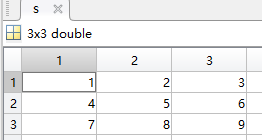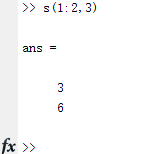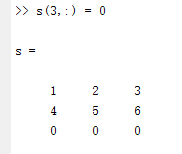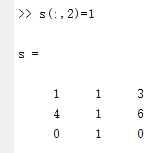• 某一行： Ki = K( i , : ); 取某一列： Kj = K( : , j ); 取某几行：Kij = K( i : j , : ); 取某几列：Kij = K( : , i : j ); i和j表示行数和列数 2. 举例分析 例如矩阵K=[1 2 3; 4 5 6 ; 7 8 9]，三行三列。 K ...
1. 操作指令
取某一行： Ki = K( i , : );
取某一列： Kj = K( : , j );
取某几行：Kij = K( i : j , : );
取某几列：Kij = K( : , i : j );

i和j表示行数和列数

2. 举例分析
例如矩阵K=[1 2 3; 4 5 6 ; 7 8 9]，三行三列。
K =[1 2 3 ;
4 5 6 ;
7 8 9];

%取K第一行
K1=K( 1 , : );
%取K第二列
K2=K( : , 2 );
%取第二三行
K23 = K( 2 : 3 , : );
%取K第一二列
K12 = K( : , 1 : 2 );

返回结果为：
>> K1

K1 =

1     2     3

>> K2

K2 =

2
5
8

>> K23

K23 =

4     5     6
7     8     9

>> K12

K12 =

1     2
4     5
7     8



展开全文• matlab对矩阵的取值赋值操作 取矩阵的几行几列，用 列表名...s(1 : 2, 3) 表示矩阵的第一行到第二行，第三列元素，即 3 6 同时，可以给对应的元素重新赋值。 s(3, : ) = 0 表示给第三行的所有元素赋值为0 ...
matlab对矩阵的取值赋值操作
取矩阵的某几行某几列，用  列表名（起始行 ： 终止行 , 起始列 ： 终止列）
取元素的一点就是，行和列之间用逗号分割开，逗号前面是行操作，逗号后面是列操作
例如：定义一个3 * 3 的矩阵ss(1 : 2, 3) 表示矩阵的第一行到第二行，第三列元素，即 3 6同时，可以给对应的元素重新赋值。
s(3, : ) = 0 表示给第三行的所有元素赋值为0s(:,2) = 1 ：给第二列所有元素赋值为1展开全文• matlab中，取出矩阵某一个值的方法如下： 1、对于二维数组： a(i, j) % 表示取出二维数组a的第 i ，第 j 列的数据 a(:, j) % 表示取出二维数组a的第 j 列的所有数据 a(i, :) % 表示取出二维数组a的第 i 的...
在matlab中，取出矩阵中某一个值的方法如下：
1、对于二维数组：
a(i, j) % 表示取出二维数组a的第 i 行，第 j 列的数据
a(:, j) % 表示取出二维数组a的第 j 列的所有数据
a(i, :) % 表示取出二维数组a的第 i 行的所有数据
2、多维数组的方法与二维数组类似（以三维数组为例）：
b(i, j, k) % 表示取出三维数组a的第k个二维数组的第 i 行，第 j 列的数据
b(:, j, k) % 表示取出三维数组a的第k个二维数组的第 j 列的所有数据
b(i, :, k) % 表示取出三维数组a的第k个二维数组的第 i 行的所有数据
b(i, j, :) % 表示取出三维数组a的每个二维数组的第 i 行，第 j 列的数据 
展开全文• 1、矩阵结构 矩阵的结构是指矩阵子元素的排列方式。 函数名称 函数功能 isempty(A) 检测矩阵是否为空 isscalar(A) ... 返回1表示矩阵某一特定类型的矩阵，返回0则表示不是。 ...
1、矩阵结构

矩阵的结构是指矩阵子元素的排列方式。

函数名称
函数功能
isempty(A)
检测矩阵是否为空
isscalar(A)
检测矩阵是否是单元素的标量矩阵
isvector(A)
检测矩阵是否是只具有一行或一列元素的一维向量
issparse(A)
检测数组是否是系数矩阵
返回1表示该矩阵是某一特定类型的矩阵，返回0则表示不是。

2、矩阵大小

包括：矩阵的维数；矩阵各维（例如最长维，用户指定的维）；矩阵元素的个数。

函数
调用格式
描述
ndims
n=ndims(X)
获取矩阵的维数
size
[m,n]=size(X)
获取矩阵各维上的长度
length
n=length(X)
获取矩阵最长维的长度
numel
n=numel(X)

获取矩阵元素的个数

size函数的返回值可以是分开显示的单个实数变量，也可以是一个行向量。

在size函数的输入参数中增加维度参数可以获取指定维度的长度，其中“1”表示行，“2”表示列

>> A=eye(5,3)

A =

1     0     0
0     1     0
0     0     1
0     0     0
0     0     0

>> ndims(A)

ans =

2

>> length(A)

ans =

5

>> [m,n]=size(A)

m =

5

n =

3

>> d=size(A)

d =

5     3

>> e1=size(A,1)

e1 =

5

>> e2=size(A,2)

e2 =

3

>> numel(A)

ans =

15

3、矩阵维度

对于空矩阵、标量矩阵、一维矩阵、二维矩阵，MATLAB都将其作为普通的二维数组对待。

需要特别注意的是：用[ ]产生的空矩阵是作为二维矩阵的。

>> a=[];b=5;c=1:3;d=magic(2);e(:,:,2)=[1,2;3,4];
>> Ndims = [ndims(a),ndims(b),ndims(c),ndims(d),ndims(e)]

Ndims =

2     2     2     2     3

4、矩阵的数据类型

矩阵作为MATLAB的内部数据储存和运算结构，其元素可以是各种各样的数据类型（数值，字符串，元胞，结构体等）

矩阵数据类型的测试函数
函数名称
函数功能
isnumeric
检测矩阵元素是否为数值型变量
isreal
检测矩阵元素是否为实数数值型变量
isfloat
检测矩阵元素是否为浮点数值型变量
isinteger
检测矩阵元素是否为整数型变量
islogical
检测矩阵元素是否为逻辑型变量
ischar
检测矩阵元素是否为字符型变量
isstruct
检测矩阵元素是否为结构体型变量
iscell

检测矩阵元素是否为元胞型变量

iscellstr
检测矩阵元素是否为结构体的元胞型变量
5、矩阵占用的内存

了解矩阵占用内存情况，对于优化MATLAB代码性能十分重要。

可以通过whos查看当前工作区中指定变量的所有信息，（变量名，矩阵大小，内存占用，数据类型）
展开全文• matlab中，取出矩阵某一个值的方法如下： 1、对于二维数组： a(i, j) % 表示取出二维数组a的第 i ，第 j 列的数据 a(:, j) % 表示取出二维数组a的第 j 列的所有数据 a(i, :) % 表示取出二维数组a的第 i ...
• 对于一个矩阵array sort(array，1); 表示矩阵array中每一列的值升序排列；   sort(array，2); 表示矩阵array中每一行的值升序排列； sortrows(array，1); 表示矩阵按第一列升序排列； ...
• 应用方式 （1）通过下标 如果给出的下标大于矩阵的行数或...end运算符：表示某一维的末尾元素下标 表示最后一行所有列的元素 表示第1行和第4行，从第3列到最后一列的元素 利用空矩阵删除矩阵的元素 删除第2列和第4
• 目录基础Array（Vector and Matrix）1.矩阵表达方式2.矩阵的乘3....1 3])就是2*2的矩阵4.Colon Operator等差数列建立矩阵去除某一行5.Array Concatentation（增广矩阵） F=[C D] F=[C;D]6.Array Man
• 例如，A是个元胞数组那么A{i，j}表示：第i第j列矩阵 A{i，j}（m，n）表示：元胞第ij列矩阵的第m第n列 转载于:https://www.cnblogs.com/zhouerba/p/7511187.html...
• 三、下标：矩阵A的第i第j列元素被表示成为：A(i,j)。即A(2,4)表示为第2第4列的元素。所以计算第四列的元素之和可以如下运算： A(1,4)+A(2,4)+A(3,4)+A(4,4)这将生成： ans= 34但是这并不是求解某一列元素之和...
• 多项式表示方法：MATLAB中，个多项式用个1n+1列的矩阵表示：[an,an-1,...，a2,a1,a0]矩阵的每个元素表示多项式每项的系数，从高次向低次排列。比如上面这个矩阵表示的多项式就是： 注意一点：如果项的...
• MATLAB中randi函数用于产生均匀分布的伪随机整数。 ...本人是在创建稀疏矩阵，需要随机填充某行某列的数据，所以用到了此函数 octave:26> randi(100,1,1) ans = 11 octave:27> randi(100)
• matlab中，取出矩阵某一个值的方法如下： 1、对于二维数组： a(i, j) % 表示取出二维数组a的第 i ，第 j 列的数据 a(:, j) % 表示取出二维数组a的第 j 列的所有数据 a(i, :) % 表示取出二维数组a的第 i 的...
• matlab 相机标定代码 摄像机标定(Camera calibration)简单来说是从世界坐标系换到图像坐标系的过程，也就是求最终的投影矩阵的过程。 基本的坐标系： 世界坐标系； 相机坐标系； 成像平面坐标系； 像素坐标系 ...
• matlab中，我们经常会用到求取一个矩阵中的某一行或者某一列的情况，这里进行一下说明：   比如矩阵A=rand(128,8) ,这表示一个128行8列的随机矩阵，我们想提取其中的第i行，就可以这么写：A（i，：），如果想...
• 如果个.m中是个整个的程序，可以直接按F5进行保存并且运行； 如果想要运行程序中的几行，按F9进行运行。 我们可以对程序进行打断点，在每的前面，F10为单步调试，可以看到每步执行的结果。 (2)matlab中的...
• 提示∶矩阵Ax中，每一行表示一个人脸区域特征向量（10个区域），每一列表示一个区域特征（22个特征），特征值范围1-100（可以随机生成）。(A1,A2,…A10,) （2）随机采集到某一个人的人脸图像，提取该人的人脸特征...人脸识别 python
• 假定该 FIS 有 N 个输入和 M 个输出，则每有 N M 2 个元素，前 N 个数分别表示 N 个输入变量的某一个语言名称的 index，没有的话用 0 表示，后面的 M 个数也类似，最后两个分别表示该条规则的权重和个条件的关系...
• 假定该 FIS 有 N 个输入和 M 个输出，则每有 N+M+2 个元素，前 N 个数分别表示 N 个输入变量的某一个语言名称的 index，没有的话用 0 表示，后面的 M 个数也类似，最后两个分别表示该条规则的权重和各个条件的关系...语言
• 比如一行数据a = [1 2 3 4]，那么如何在4的末尾添加一个5呢？利用方括号进行矩阵拼接可以解决这个问题。请看下面的例子： a = [1 2 3 4 ]; % 已有行向量 >> % >>表示实时运行结果 a = 1 2 3 4 a = [a...
• % pr: pr是一个R×2的矩阵,R为感知器中输入向量的维度(本例中使用35个字符表征一个字母，那么其维度为35),每一行表示输入向量每个分量的最小值和最大值。在本例中只有0和1. % T: T表示输出节点的个数,标量（本例...
• 二维相位解包裹 根据Itoh方法，可以将一维相位解包裹推广到二...选取相位图中的某一行（列）作为起始行（列），遍历相位图的每一行（列），比较相邻两个点的相位值，若相邻两点的相位差大于pi，则后一个点的相位加...
• 题目： 公司在六个城市 c 1 , c 2 , L , c 6 中有分公司,从 c i 到 c j 的直接航程票价记在 ...1.在第一行找从出发点c1到各个目标点的距离，并把距离最小的点记下（该题为城市c6最小，距离为1...
• 当未知结点接收到来自不同锚点的分组信息数量超过某一门限或在一定接收时间之后，就可以计算这些锚点所组成的多边形的质心，作为确定出自身位置。由于质心算法完全基于网络连通性，无需锚点和未知结点之间的协作和...
• wrcoef2 由多层小波分解重构某一层的分解信号 upcoef2 由多层小波分解重构近似分量或细节分量 detcoef2 提取二维信号小波分解的细节分量 appcoef2 提取二维信号小波分解的近似分量 upwlev2 二维小波分解的单层...
• 众所周知，MATLAB拥有强大的矩阵运算能力，灵活多变的外部应用程序编程接口，作为矩阵数值运算的首选工具具有无可比拟的强大优势，但是其体积过于庞大，作为仿MATLAB个简化软件Scilab因此诞生。而在符号运算中...
• temp = X1**(i-j)*(X2**j) #矩阵直接乘相当于matlab中的点乘.* out = np.hstack((out, temp.reshape(-1,1))) return out 6、使用scipy的优化方法 梯度下降使用scipy中optimize中的fmin_bfgs函数 调用scipy中的...# matlab表示矩阵某一行matlab 订阅